# 玩的就是心跳 —— 使用 PulseSensor 脉搏传感器测量心率

Posted by Shao Guoji on June 29, 2017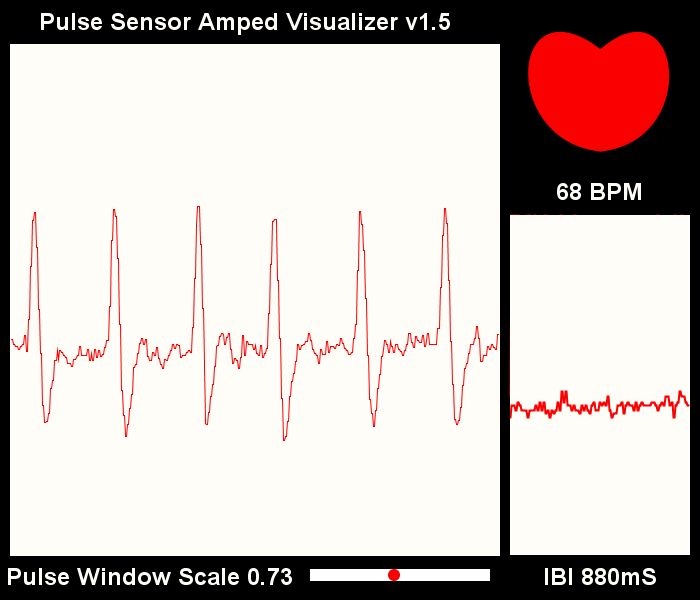### PulseSensor 脉搏传感器介绍

#### 功能原理

PulseSensor 是一款用于脉搏心率测量的光电反射式模拟传感器。将其佩戴于手指、耳垂等处，利用人体组织在血管搏动时造成透光率不同来进行脉搏测量。传感器对光电信号进行滤波、放大，最终输出模拟电压值。单片机通过将采集到的模拟信号值转换为数字信号，再通过简单计算就可以得到心率数值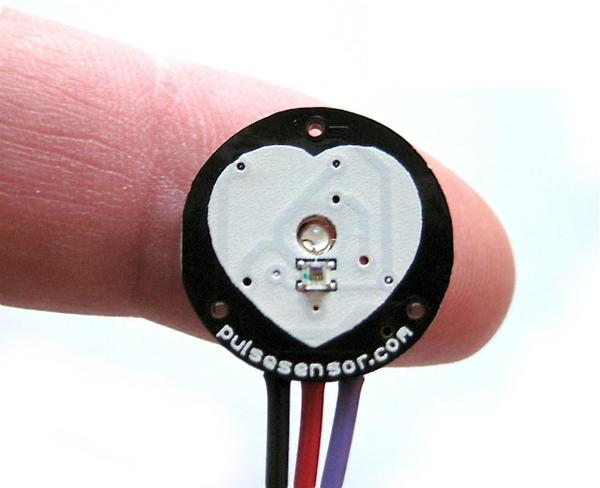PulseSensor 是一款开源硬件，目前国外官网上已有其对应的开源 arduino 程序上位机 Processing 程序，其适用于心率方面的科学研究和教学演示，也非常适合用于二次开发。 网上关于传感器的 arduino 资料已经十分丰富（毕竟同为开源硬件），本文采用 STM32F407系列芯片 的 ADC 模块读取并处理传感器数据，实现心率测量。

#### 引脚定义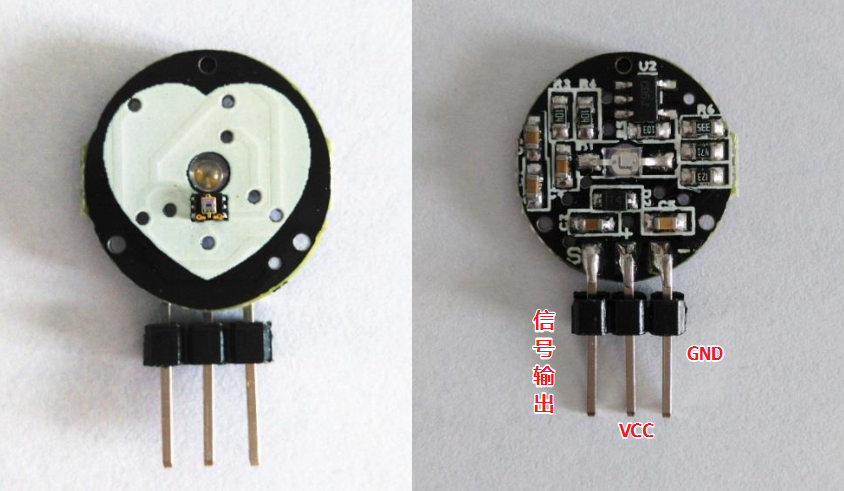### 读取传感器电压值 —— STM32 ADC 功能配置

#### 硬件配置

PA2 S
3V3 +
GND -

``````/******************** ADC通道2初始化函数 ************************/
{
RCC->AHB1ENR |= 1 << 0;
GPIOA->MODER &= ~(3 << (2 * 2));
GPIOA->MODER |= 3 << (2 * 2);

RCC->APB2ENR |= 1 << 8;     //使能ADC模块时钟
ADC1->CR1 &= ~(3 << 24);    //12位分辨率
ADC1->CR1 &= ~(1 << 8);     //非扫描模式
ADC1->CR2 &= ~(3 << 28);    //禁止外部触发
ADC1->CR2 &= ~(1 << 11);    //右对齐
ADC1->CR2 &= ~(1 << 1);     //单次转换
ADC->CCR |= 2 << 16;        //6分频
ADC1->SMPR2 |= 0x07 << 6;   //480采样周期
ADC1->SQR1 &= ~(0x0f << 20); //1次转换
ADC1->SQR3 |= 0x02 << 0;     //转换的通道为通道2

}
``````

``````/******************** ADC通道2转换函数 ************************/
{
u8 i,j;
u16 buff = {0};
u16 temp;

for(i = 0; i < 10; i ++)
{
/* 开始转换 */

/* 等待转换结束 */
while( !(ADC1->SR & (1 << 1)) )
{
/* 等待转换接收 */
}
}

/* 把读取值的数据按从小到大的排列 */
for(i = 0; i < 9; i++)
{
for(j = 0 ; j < 9-i; j++)
{
if(buff[j] > buff[j+1])
{
temp = buff[j];
buff[j] = buff[j+1];
buff[j+1] = temp;
}
}
}

/* 求平均值 */
temp = 0;
for(i = 1; i < 9; i++)
{
temp += buff[i];
}

return temp/8;
}
``````

#### 串口打印，验证数据读取

``````void Print_Wave(void)
{
int temp, i;

temp = Get_ADC_1_CH2() / 20;   // 缩小一个倍数

for (i = 0; i < temp; i++)
printf("*");

printf ("\r\n");
}
``````

``````int main(void)
{
Usrat_1_Init(84,115200,0);
Timer_6_Init();

while(1)
{
Print_Wave();
Timer_6_Delay_ms(5);  // 延时 5 ms
}
}
``````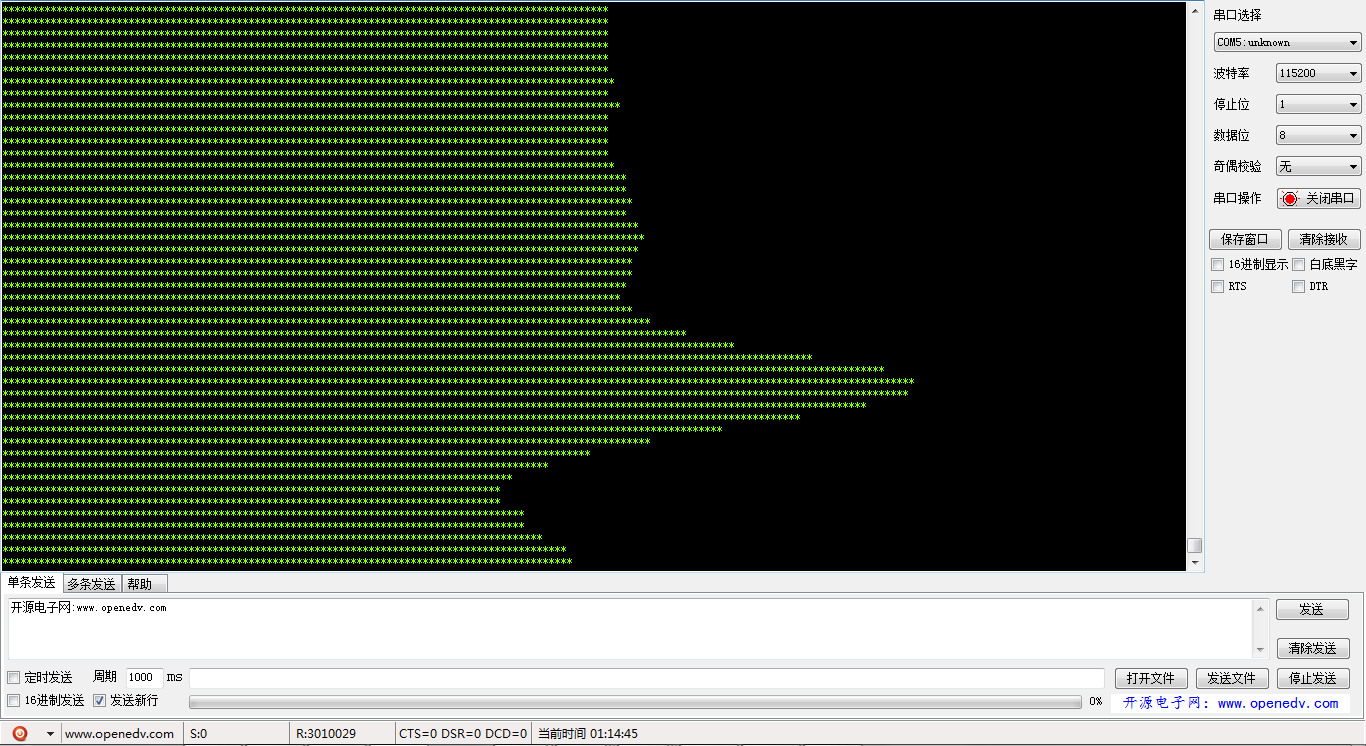### 计算心率值 —— 采样数据处理算法

IBI： 相邻两次脉搏的时间间隔（单位：ms） BPM(beats per minute)：心率，一分钟内的心跳次数

#### 核心操作 —— 识别一个脉搏信号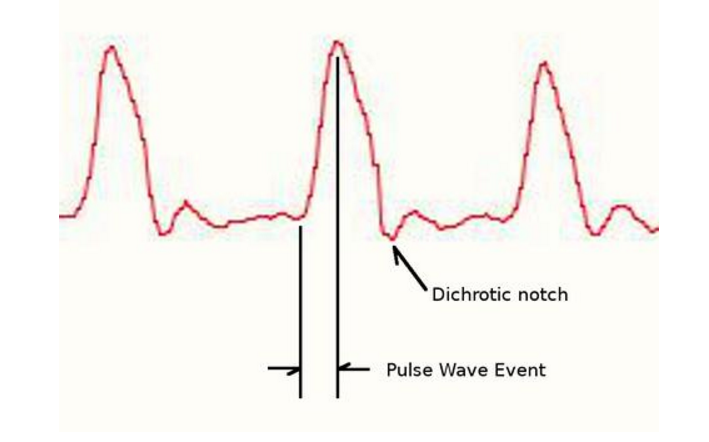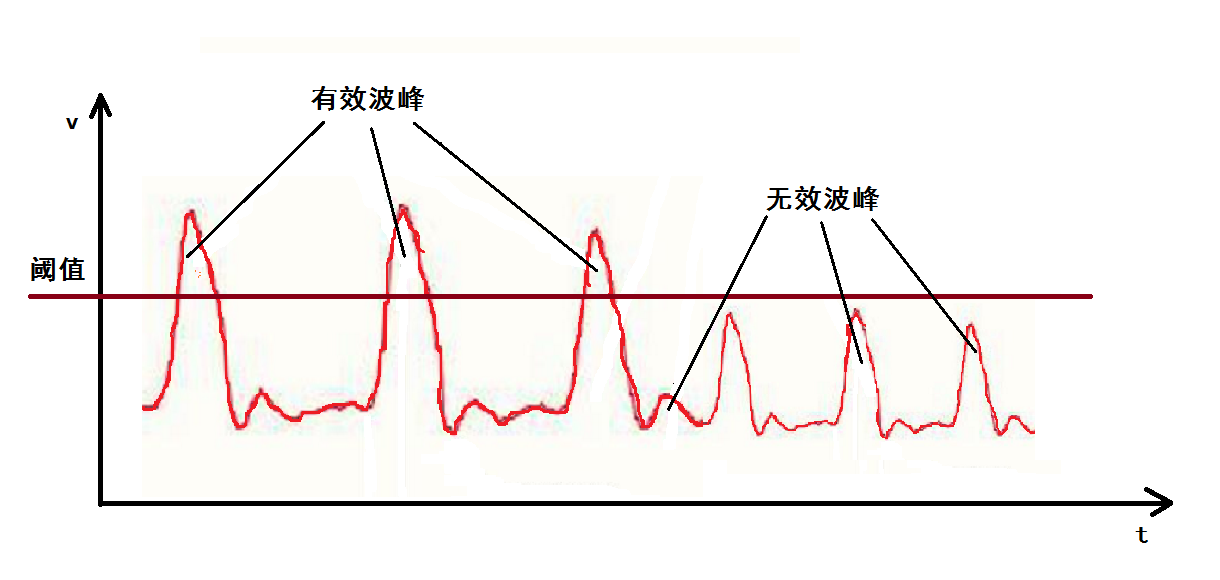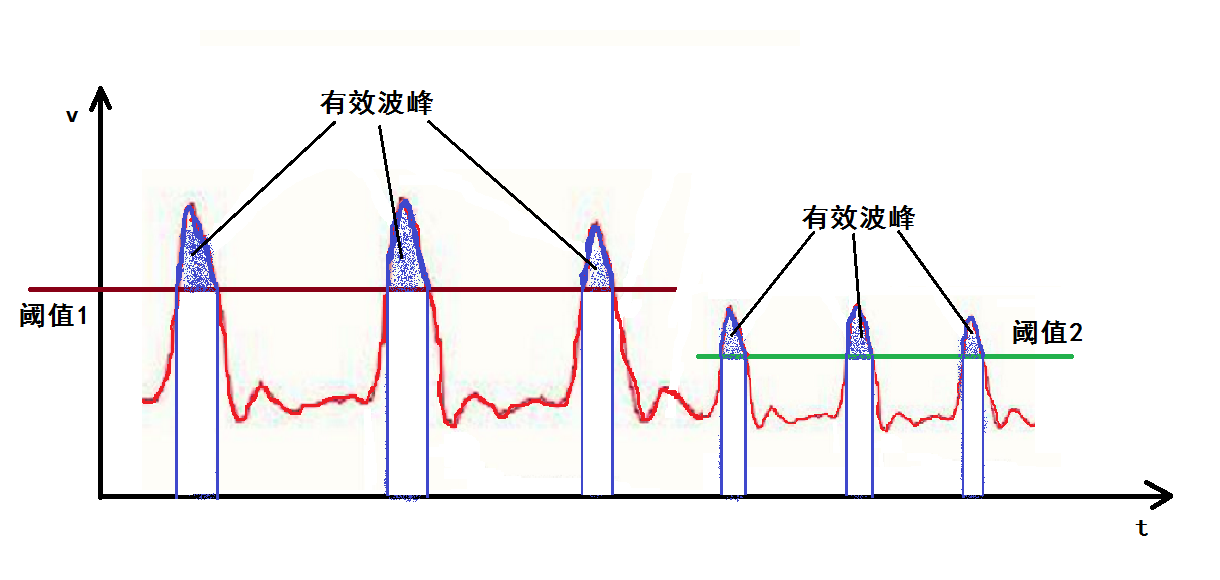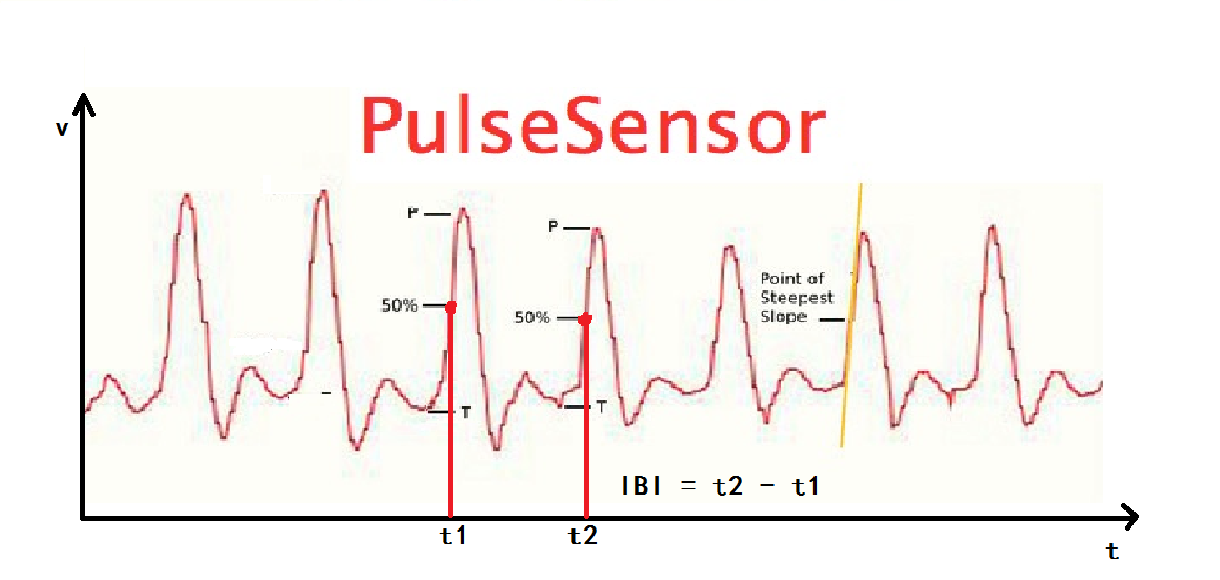#### 算法整体框架与代码实现

1. 缓存一个波形周期内的多次采样值，求出最大最小值，计算出振幅中间值作为信号判定阈值
2. 通过把当前采样值和上一采样值与阈值作比较，寻找到「信号上升到振幅中间位置」的特征点，记录当前时间
3. 寻找下一个特征点并记录时间，算出两个点的时间差值，即相邻两次脉搏的时间间隔 IBI
4. 由 IBI 计算心率值 BPM

``````int main(void)
{
Usrat_1_Init(84,115200,0);
Timer_6_Init();

while(1)
{
//Print_Wave();

if (index >= BUFF_SIZE)
{
index = 0;	// 数组填满，从头再填

// 通过缓存数组获取脉冲信号的波峰、波谷值，并计算中间值作为判定参考阈值
max = Get_Array_Max(data, BUFF_SIZE);
min = Get_Array_Min(data, BUFF_SIZE);
mid = (max + min)/2;
filter = (max - min) / 2;
}

PRE_PULSE = PULSE;	// 保存当前脉冲状态
PULSE = (readData > mid) ? TRUE : FALSE;  // 采样值大于中间值为有效脉冲

if (PRE_PULSE == FALSE && PULSE == TRUE)  // 寻找到「信号上升到振幅中间位置」的特征点，检测到一次有效脉搏
{
pulseCount++;
pulseCount %= 2;

if(pulseCount == 1) // 两次脉搏的第一次
{
firstTimeCount = timeCount;   // 记录第一次脉搏时间
}
if(pulseCount == 0)  // 两次脉搏的第二次
{
secondTimeCount = timeCount;  // 记录第二次脉搏时间
timeCount = 0;

if ( (secondTimeCount > firstTimeCount))
{
IBI = (secondTimeCount - firstTimeCount) * SAMPLE_PERIOD;	// 计算相邻两次脉搏的时间，得到 IBI
BPM = 60000 / IBI;  // 通过 IBI 得到心率值 BPM

if (BPM > 200)    //限制BPM最高显示值
BPM = 200;
if (BPM < 30)    //限制BPM最低显示值
BPM=30;
}

}

printf("SIG = %d IBI = %d, BMP = %d\r\n\r\n", readData, IBI, BPM);  // 串口打印调试
//          printf("B%d\r\n", BPM);  // 上位机B数据发送
//          printf("Q%d\r\n", IBI);  // 上位机Q数据发送
}

SIG = readData - 1500;  // 脉象图数值向下偏移，调整上位机图像

//      printf("S%d\r\n", SIG);  // 上位机S数据发送

timeCount++;  // 时间计数累加
Timer_6_Delay_ms(SAMPLE_PERIOD);  // 延时再进行下一周期采样
}
}
``````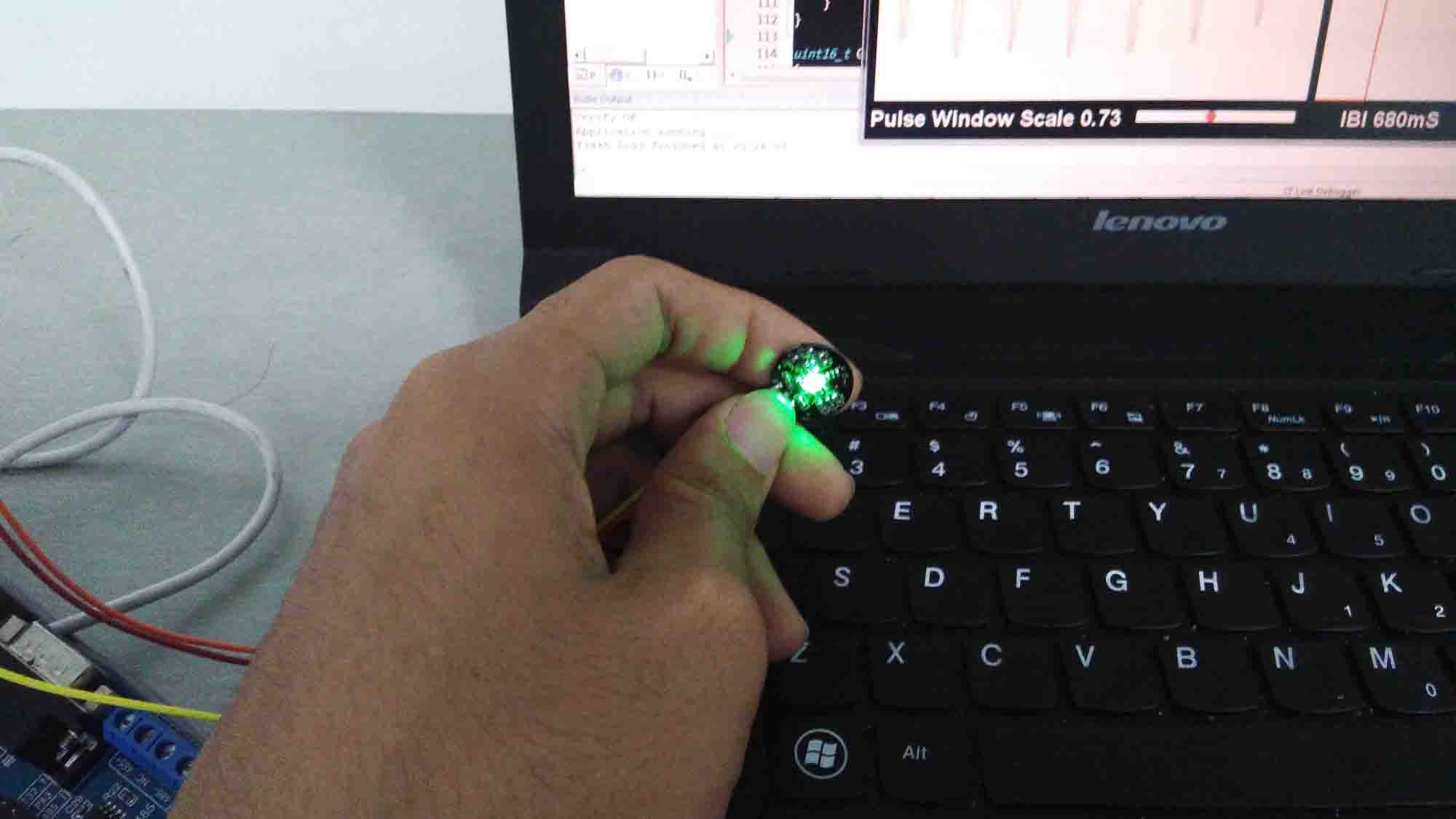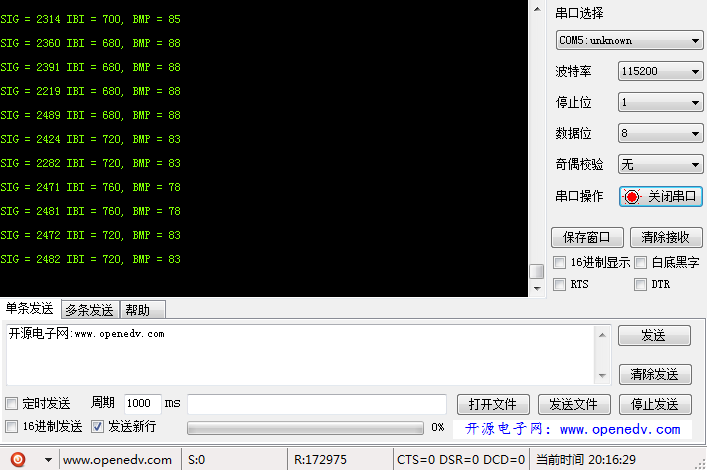1. 避免手指触碰传感器背面
2. 传感器与手指之间不要施加过大压力，否则会阻碍血液流动而读不到脉搏信号
3. 传感器与手指之间的接触要保持稳定，按压力度的轻微变化都会影响电压值

### 使用 Processing 上位机查看心率

PulseSensor 官方提供了 Processing 语言编写的、超好看的上位机软件，而且项目在 Github 上开源，通过上位机可以查看实时心率图、心率值 BPM 和 脉搏间隔 IBI，那要如何使用这么好用的软件呢？

#### 修改串口数据发送格式#### 下载 Processing 上位机软件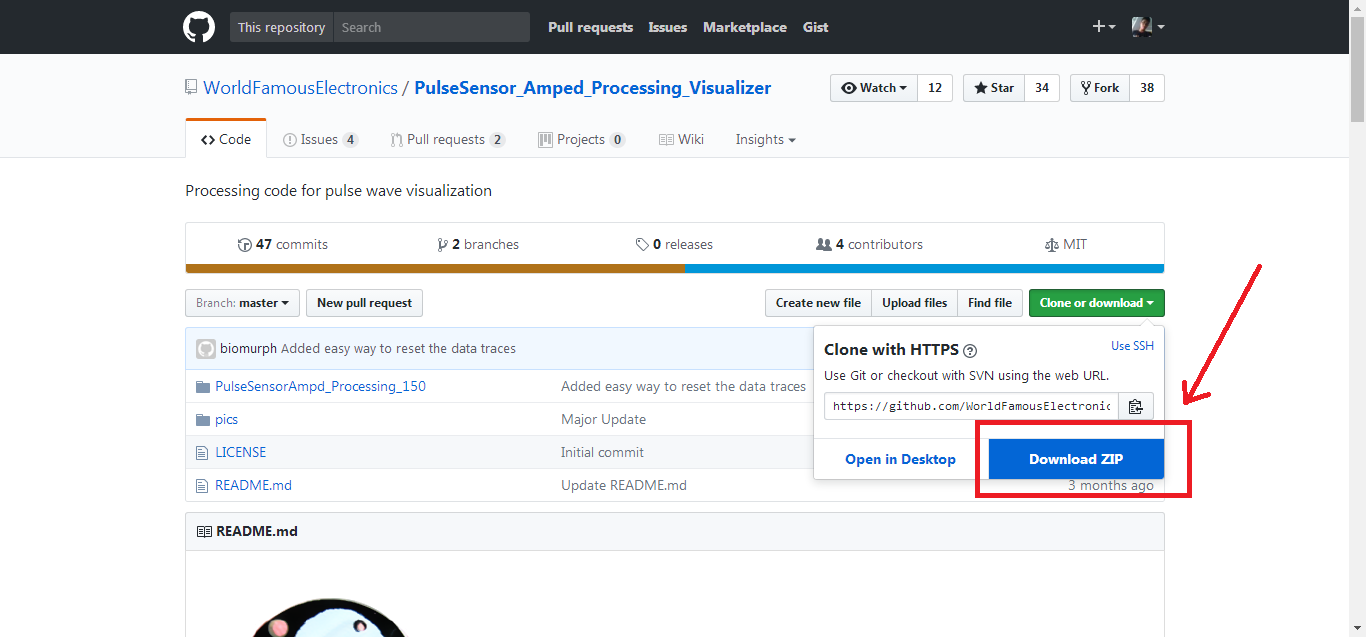#### 下载 Processing 运行环境、打开上位机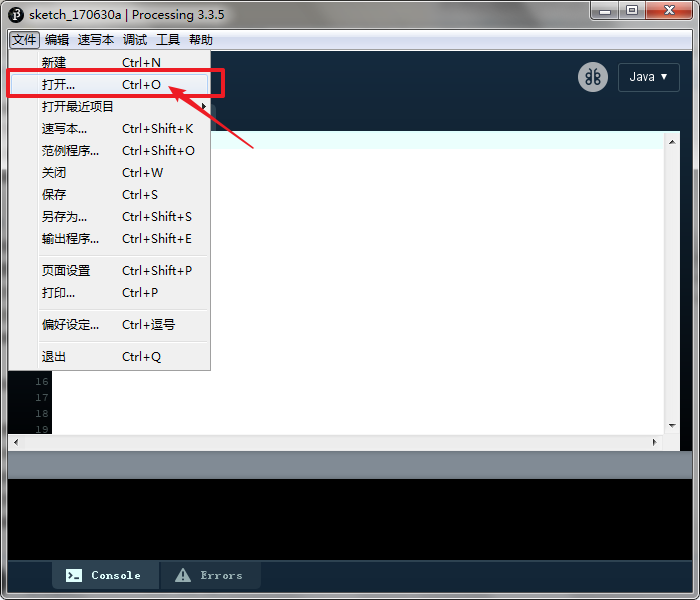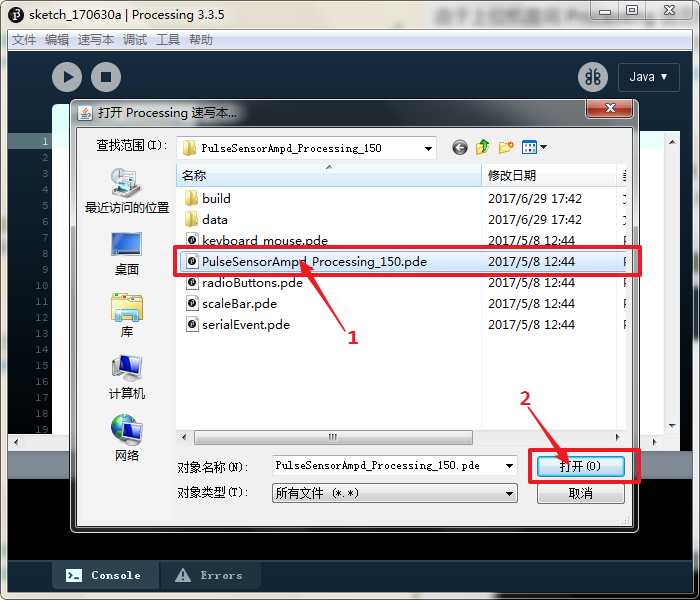#### 运行上位机，显示心率值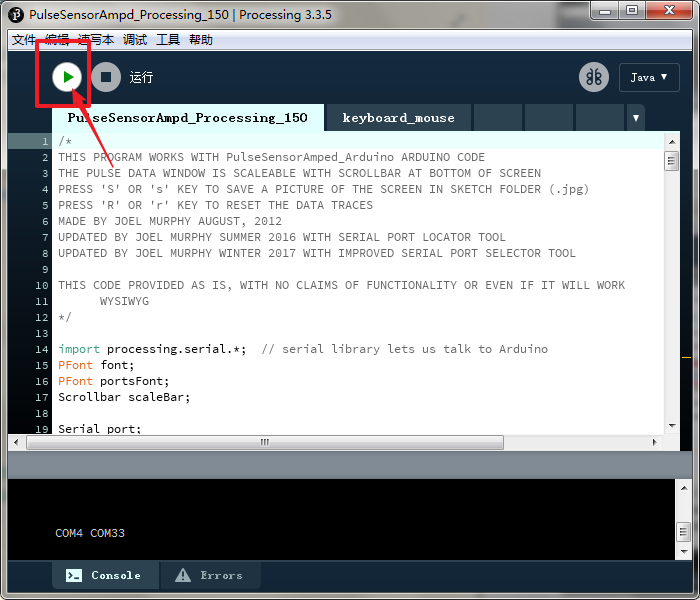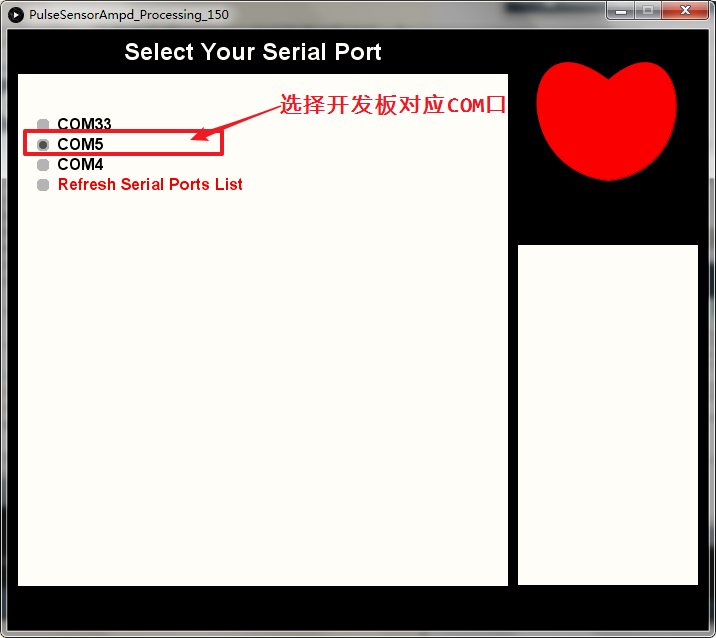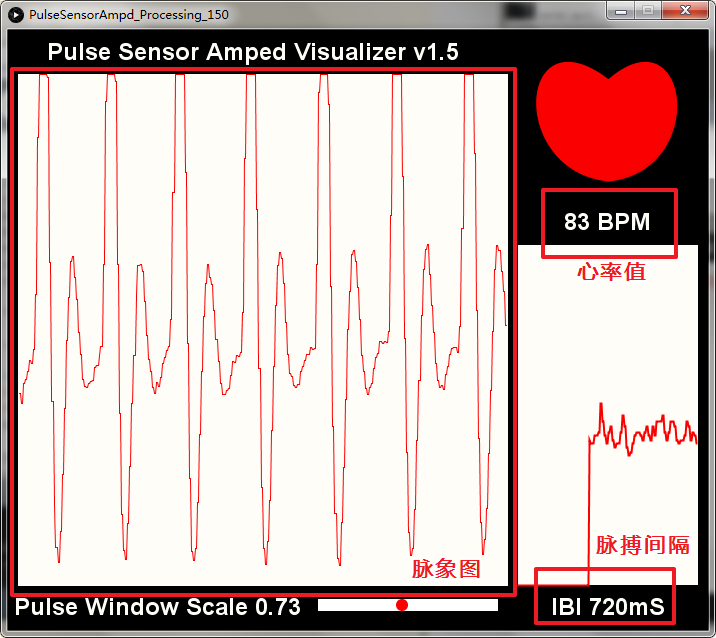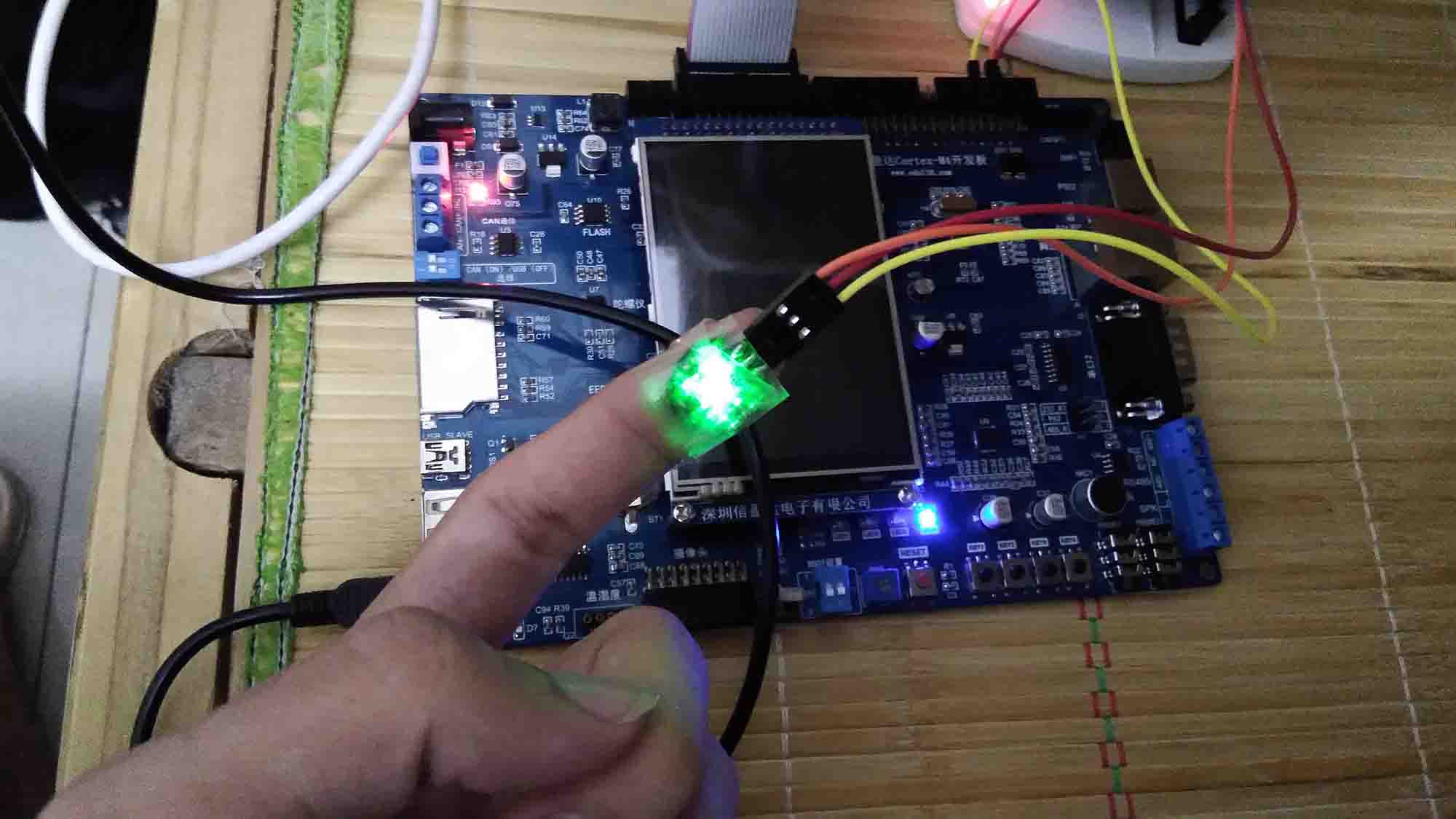### 总结

keil 工程源码下载：测心率_脉搏传感器 密码 8ut8